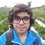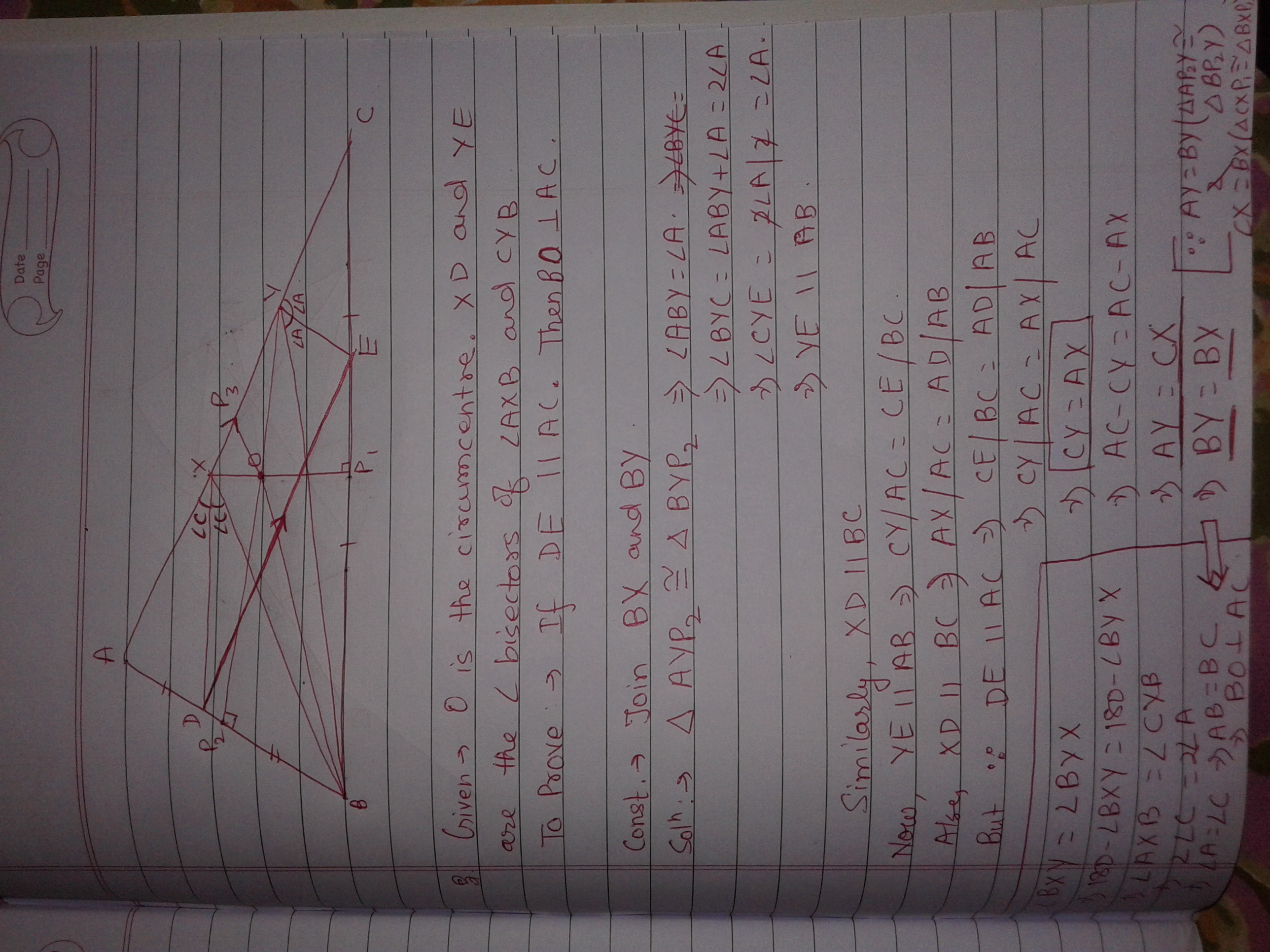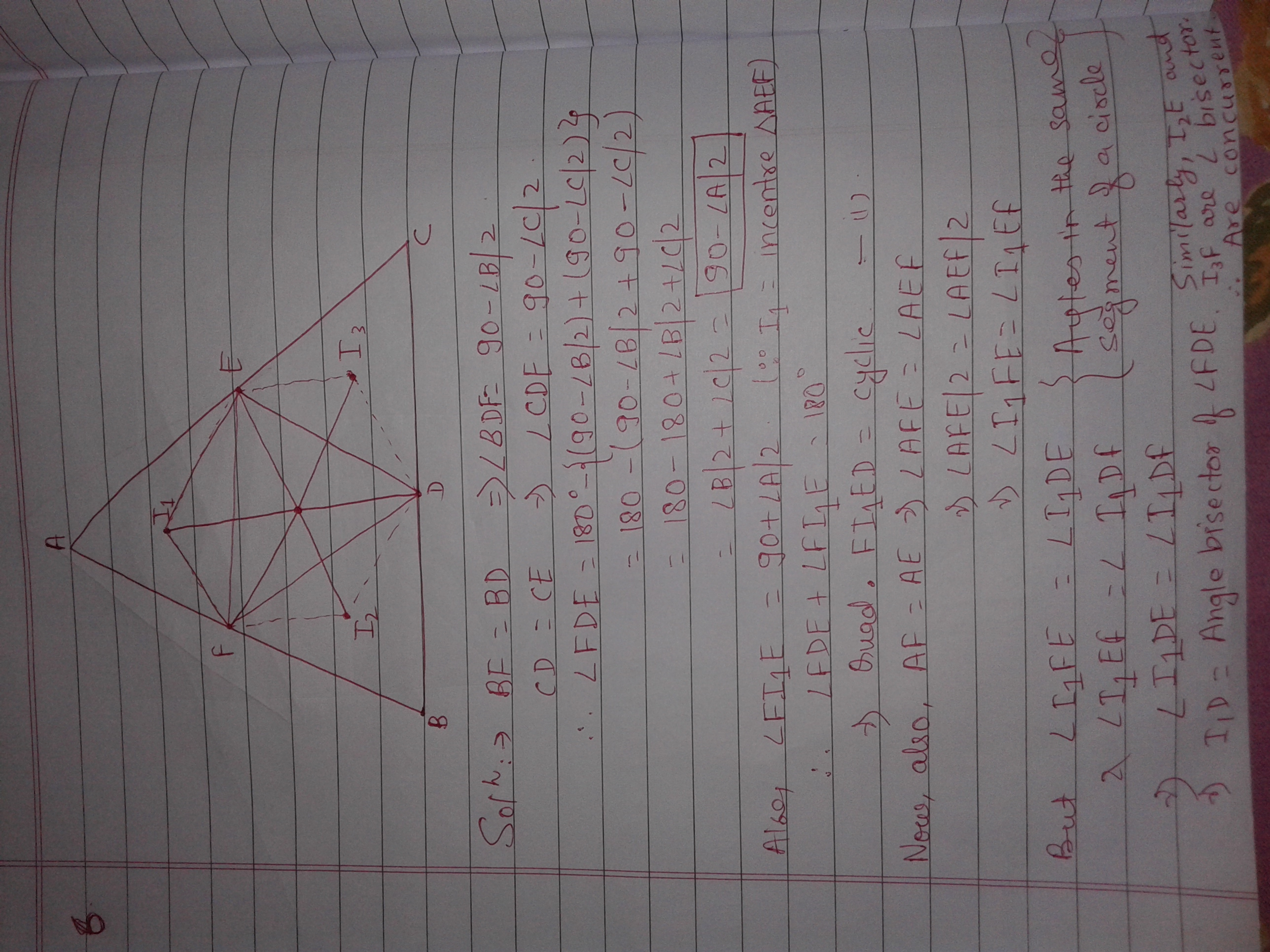# RMO 2014 Mumbai Region

Regional Mathematical Olympiad 2014 (Mumbai Region)

Instructions

• There are six questions in this paper. Answer all questions.
• Each question carries 10 points
• Use of protractors, calculators, mobile phone is forbidden.
• Time alloted: 3 hours

Questions

1 Three positive real numbers $a, b, c$ are such that $a^2 + 5b^2 + 4c^2 - 4ab - 4bc = 0$. Can $a, b, c$ be the lengths of sides of a triangle? Justify your answer.

2 The roots of the equation

$x^3 -3ax^2 + bx + 18c = 0$

form a non-constant arithmetic progression and the roots of the equation

$x^3 + bx^2 + x - c^3 = 0$

form a non-constant geometric progression. Given that $a, b, c$ are real numbers, find all positive integral values $a$ and $b$.

3 Let $ABC$ be an acute-angled triangle in which $\angle ABC$ is the largest angle. Let $O$ be its circumcentre. The perpendicular bisectors of $BC$ and $AB$ meet $AC$ at $X$ and $Y$ respectively. The internal bisectors of $\angle AXB$ and $\angle BYC$ meet $AB$ and $BC$ at $D$ and $E$ respectively. Prove that $BO$ is perpendicular to $AC$ if $DE$ is parallel to $AC$.

4 A person moves in the $x-y$ plane moving along points with integer co-ordinates $x$ and $y$ only. When she is at point $(x,y)$, she takes a step based on the following rules:

(a) if $x+y$ is even she moves either to $(x+1,y)$ or $(x+1, y+1)$;

(b) if $x+y$ is odd she moves either to $(x,y+1)$ or $(x+1, y+1)$.

How many distinct paths can she take to go from $(0,0)$ to $(8,8)$ given that she took exactly three steps to right $((x,y)$ to $(x+1,y))$?

5 Let $a, b, c$ be positive numbers such that

$\frac{1}{1+a} + \frac{1}{1+b} + \frac{1}{1+c} \leq 1.$

Prove that $(1+a^2 )(1+b^2)(1+c^2) \geq 125$. When does the equality hold?

6 Let $D, E, F$ be the points of contact of the incircle of an acute-angled triangle $ABC$ with $BC, CA, AB$ respectively. Let $I_1, I_2, I_3$ be the incenters of the triangles $AFE, BDF, CED,$ respectively. Prove that the lines $I_1D, I_2E, I_3F$ are concurrent.

Post your innovative solutions below!! Enjoy!!!!!!!!Note by Pranshu Gaba
6 years, 7 months ago

This discussion board is a place to discuss our Daily Challenges and the math and science related to those challenges. Explanations are more than just a solution — they should explain the steps and thinking strategies that you used to obtain the solution. Comments should further the discussion of math and science.

When posting on Brilliant:

• Use the emojis to react to an explanation, whether you're congratulating a job well done , or just really confused .
• Ask specific questions about the challenge or the steps in somebody's explanation. Well-posed questions can add a lot to the discussion, but posting "I don't understand!" doesn't help anyone.
• Try to contribute something new to the discussion, whether it is an extension, generalization or other idea related to the challenge.

MarkdownAppears as
*italics* or _italics_ italics
**bold** or __bold__ bold
- bulleted- list
• bulleted
• list
1. numbered2. list
1. numbered
2. list
Note: you must add a full line of space before and after lists for them to show up correctly
paragraph 1paragraph 2

paragraph 1

paragraph 2

[example link](https://brilliant.org)example link
> This is a quote
This is a quote
    # I indented these lines
# 4 spaces, and now they show
# up as a code block.

print "hello world"
# I indented these lines
# 4 spaces, and now they show
# up as a code block.

print "hello world"
MathAppears as
Remember to wrap math in $$ ... $$ or $ ... $ to ensure proper formatting.
2 \times 3 $2 \times 3$
2^{34} $2^{34}$
a_{i-1} $a_{i-1}$
\frac{2}{3} $\frac{2}{3}$
\sqrt{2} $\sqrt{2}$
\sum_{i=1}^3 $\sum_{i=1}^3$
\sin \theta $\sin \theta$
\boxed{123} $\boxed{123}$

Sort by:

Did anyone get the proofs for question5 &6

- 6 years, 7 months ago

There are 2 ways to solve this. 1. AM-GM inequality. 2. Without using any theorems, we can prove the square of each individual term to be greater than 4(try figuring out on your own first) and then add 1 to each term. Finally multiply all the terms to get the final result. Equality holds true at a=b=c=2(which is clearly visible)

- 6 years, 7 months ago

Positive integer solution of 1/a+1/b+1/c+1/d =1

- 5 years, 9 months ago

whats the answer for 4th question

- 6 years, 7 months ago

I don't have the official answers right now, but when I solved it I got 462 distinct paths. (I may be wrong)

- 6 years, 7 months ago

How ???

- 6 years, 7 months ago

Here's one way to start. We make a graph of all the possible paths. It looks something likeimage

According to the condition in the question, the person must step on exactly 3 of the red arrows. Can you continue now?

- 6 years, 7 months ago

i guess the person could step on the red arrows 5 times

- 6 years, 7 months ago

How ? The question states that the person moves right exactly three times, so three red arrows.

- 6 years, 7 months ago

my bad. i thought the red arrows were the ones when he goes diagonally. he can go diagonally five times. sorry.

- 6 years, 7 months ago

I would say that 462 sounds right.Since the person is allowed to go only 3 steps to the right, she can go only 3 units on the x-axis. And since she needs to reach 8 on the x axis, she must take exactly 5 steps to reach the required point. But now having only options as going upwards and moving diagonally, she needs exactly 5 diagonals as that is the only other step which can take her +1 unit on both axes. But moving right doesn't contibute to moving upwards. And since the 5 diagonal steps contribute only 5 steps upwards, she must take exactly 3 steps upwards. Now fixing the diagonal steps will complementarily fix the steps upwards and towards the right or vice versa. So, the required answer is 462 or 11C5

- 6 years, 7 months ago

or you can do this. if you select 5 diagonals out of the possible diagonal paths, there exists a unique path.

- 6 years, 7 months ago

- 6 years, 7 months ago

why 11C5

- 6 years, 7 months ago

5th is so easy. Basic CS/AM-HM.

- 6 years, 7 months ago

1 and 5 were so easy. I wonder why they asked them. I was on the right track for the 2nd question however, a shitty mistake while writing the equation led to me getting no solutions :-( I also attempted the 4 and the 6th but I am not sure of the solutions. How much marks do you expect? What is the expected cut-off?

- 6 years, 7 months ago

The cutoff must be somewhere around 40.

- 6 years, 7 months ago

Did you get the 3rd one? I tried but I could not get anywhere in that problem.

- 6 years, 7 months ago

No, I tried but I didn't get it.

- 6 years, 7 months ago

in q3 triangle abc is isoceles can be proved.so the perpendicular bisector of side ac is a cevian so BO perpendicular to AC

- 6 years, 7 months ago

i am selected for inmo

- 6 years, 7 months ago

I am preparing for RMO and I am in class 10 please someone help me in preparing.and pls help me in the 2nd Question i tried but i dont know where i am wrong

- 6 years, 5 months ago

Pranshu, can you tell me which latex you used to separate the questions by a horizontal line?

- 5 years, 7 months ago

 1 --- 

Priyanshu, this is not latex; it is markdown. Enter three hyphens (shown above) in a new line to get a horizontal line.

- 5 years, 7 months ago

What is the meaning of a non constant arithmetic and geometric progression

- 4 years, 9 months ago

Well the Maharastra is much ahead in the race for IMO in India. I tried the geometry ones(nice). Both Mumbai and Pune are doing well. I couldn't try the non geometric one due to lack of time. Here are the geometric ones.- 4 years, 5 months ago

Nicely done :)

- 4 years, 5 months ago

Ya really a nice solution.Thumbs Up to you.

- 2 years, 11 months ago

6 is easy

- 3 years, 12 months ago

what is the ans to question 2 i m getting a =2 b=9 there could be more values

- 6 years, 7 months ago

$a=2$ and $b=9$ are the only positive integer solutions.

- 6 years, 7 months ago

ya even i got that. and for question 1 i got that a,b and c can never form the sides of a triangle.

- 6 years, 7 months ago

a, b, c are in ratio 4:2:1, hence they cannot be the sides of a triangle.

- 6 years, 7 months ago

yes. i got the same. how did you do it? i factorized the equation.

- 6 years, 7 months ago

I made perfect squares. The equation becomes $(a-2b)^2 + (b-2c)^2 = 0$.

- 6 years, 7 months ago

how many did you get?

- 6 years, 7 months ago

1, 2 and 4. Which ones did you get?

- 6 years, 7 months ago

i too got 1,2 and 4 but i am not sure about my answer to the fourth question.

- 6 years, 7 months ago

Did you attend Pace in grade 9 and 10? I have a feeling that we have studied together in the "fast-track" batch lectures held in dadar.

- 6 years, 7 months ago

i attended PACE in 9th an 10th and even attended the fast-track batch lectures.

- 6 years, 7 months ago

Positive integer solution of 1/a+1/b+1/c+1/d =1

- 5 years, 9 months ago